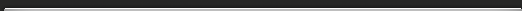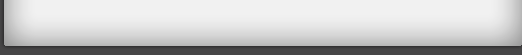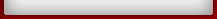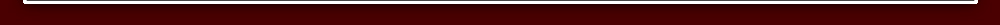Tutorial 7

## Tutorial 7

Method for dividing by 9.
23 / 9 = 2 remainder 5
The first figure of 23 is 2, and this is the answer.
The remainder is just 2 and 3 added up!
43 / 9 = 4 remainder 7
The first figure 4 is the answer
and 4 + 3 = 7 is the remainder - could it be easier?

Exercise 1a Tutorial 7
Divide by 9:

1) 61 / 9 = ______ remainder ______
2) 33 / 9 = ______ remainder ______
3) 44 / 9 = ______ remainder ______
4) 53 / 9 = ______ remainder ______
5) 80 / 9 = ______ remainder ______

134 / 9 = 14 remainder 8
The answer consists of 1,4 and 8.
1 is just the first figure of 134.
4 is the total of the first two figures 1+ 3 = 4,
and 8 is the total of all three figures 1+ 3 + 4 = 8.

Exercise 1b Tutorial 7
Divide by 9:

6) 232 = ______ remainder ______
7) 151 = ______ remainder ______
8) 303 = ______ remainder ______
9) 212 = ______ remainder ______
10) 2121 = ______ remainder ______

842 / 9 = 812 remainder 14 = 92 remainder 14
Actually a remainder of 9 or more is not usually permitted because we are trying to find how many 9's there are in 842.
Since the remainder, 14 has one more 9 with 5 left over the final answer will be 93 remainder 5

Exercise 2 Tutorial 7
Divide these by 9:

1) 771 / 9 = ______ remainder ______
2) 942 / 9 = ______ remainder ______
3) 565 / 9 = ______ remainder ______
4) 555 / 9 = ______ remainder ______
5) 2382 / 9 = ______ remainder ______
6) 7070 / 9 = ______ remainder ______